Homework Help Question & Answers

# 5. Draw the hash table that results using the hash function: h(k)=kmod13 to hash the keys...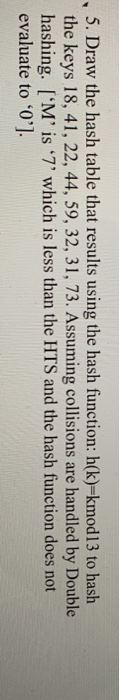5. Draw the hash table that results using the hash function: h(k)=kmod13 to hash the keys 18, 41, 22, 44, 59, 32, 31, 73. Assuming collisions are handled by Double hashing. ['M' is '7' which is less than the HTS and the hash function does not evaluate to '0'].

#### Homework Answers

Answer #1

Hey Guys! pls do like my answer and if you have any doubt then do let me know...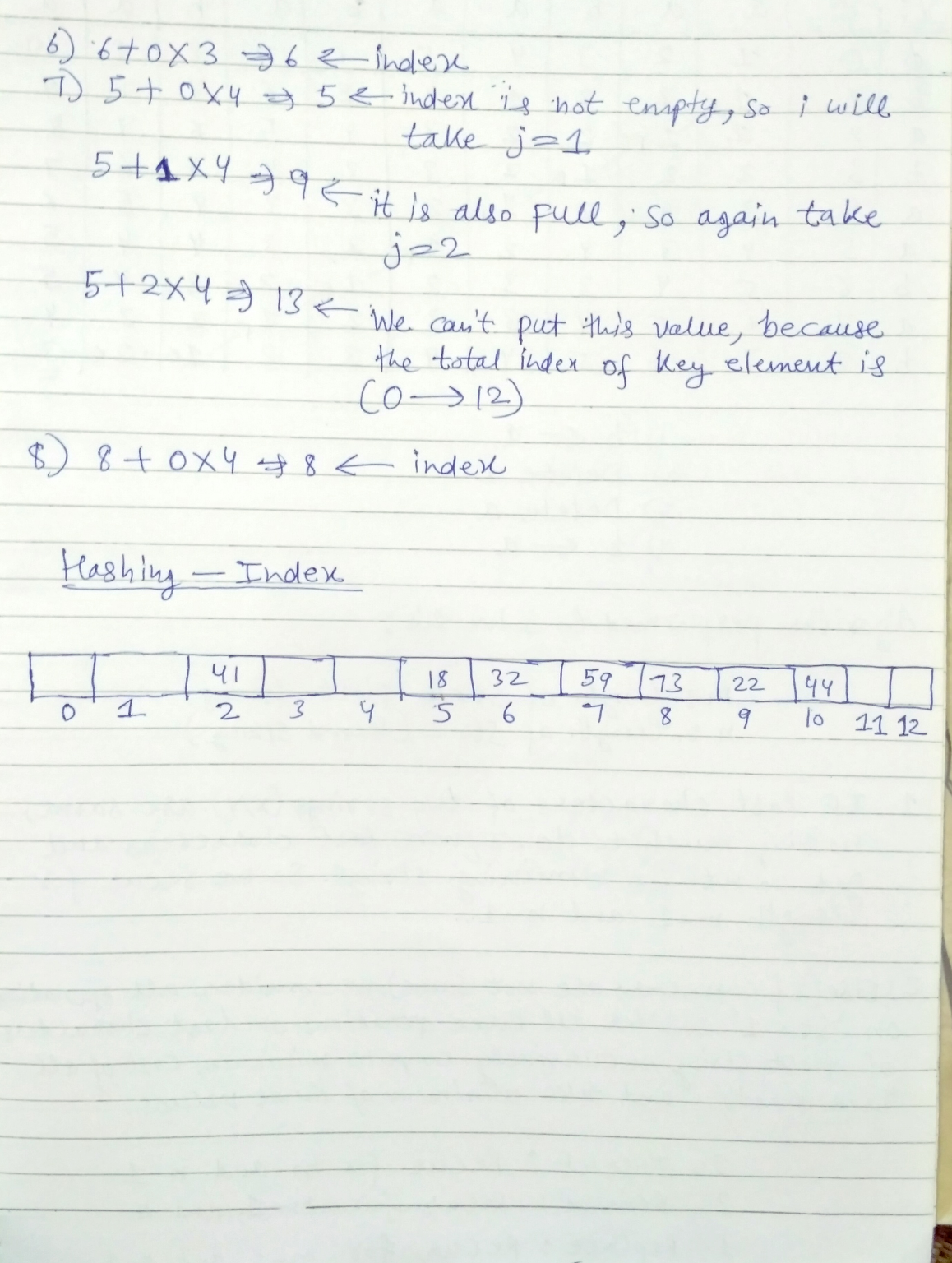Know the answer?
Your Answer:

#### Post as a guest

Your Name:

What's your source?

#### Earn Coin

Coins can be redeemed for fabulous gifts.

Not the answer you're looking for? Ask your own homework help question. Our experts will answer your question WITHIN MINUTES for Free.
Similar Homework Help Questions
• ### 3. Given input (89, 18, 49, 58, 69), h)k(mod 10) g) Iymod 8), and a hash function f(k) h(k) +j-g(...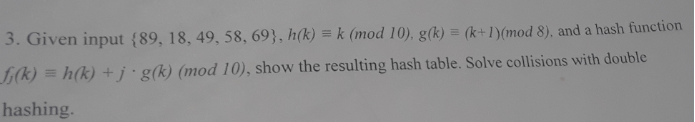3. Given input (89, 18, 49, 58, 69), h)k(mod 10) g) Iymod 8), and a hash function f(k) h(k) +j-g(k) (mod 10), show the resulting hash table. Solve collisions with double hashing. 3. Given input (89, 18, 49, 58, 69), h)k(mod 10) g) Iymod 8), and a hash function f(k) h(k) +j-g(k) (mod 10), show the resulting hash table. Solve collisions with double hashing.

• ### I need help for Q11 Please if you can, answer this question too. I need B...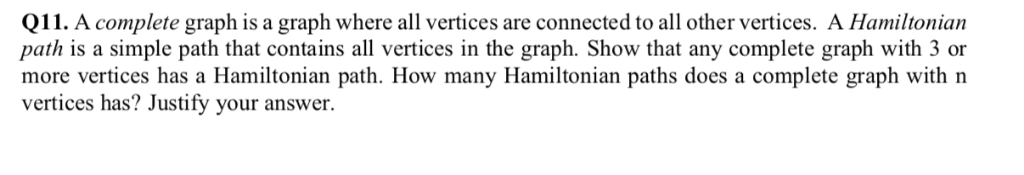I need help for Q11 Please if you can, answer this question too. I need B Q11. A complete graph is a graph where all vertices are connected to all other vertices. A Hamiltonian path is a simple path that contains all vertices in the graph. Show that any complete graph with 3 or more vertices has a Hamiltonian path. How many Hamiltonian paths does a complete graph with n vertices has? Justify your answer Q1. Draw thee 13-entry hash...

• ### Assume data is to be stored in a hash table using the following keys: 62,77.26, 42,...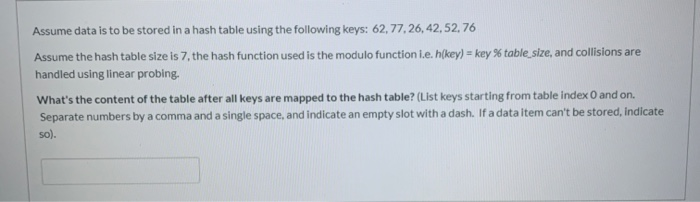Assume data is to be stored in a hash table using the following keys: 62,77.26, 42, 52,76 Assume the hash table size is 7, the hash function used is the modulo function i.e. h/key) = key % table_size, and collisions are handled using linear probing. What's the content of the table after all keys are mapped to the hash table? (List keys starting from table index 0 and on Separate numbers by a comma and a single space, and indicate...

• ### DATA STRUCTURE: Draw the structure that results from inserting the following numerical keys into each of...

DATA STRUCTURE: Draw the structure that results from inserting the following numerical keys into each of the structures given, starting from an empty structure. Keys: 17 29 25 48 9 36 3 31 (a) Heap (min heap) (b) Hash table of size 11, using double hashing with primary hash function h(x) =x mod 11 and secondary hash function d(x) = 5 − (x mod 5) (c) AVL Tree

• ### 10. Submission In this question you will work with a hash table that uses double hashing. The hash table is size 11, the primary hash function is h(K)-K mod 11, and the secondary hash function is hp(...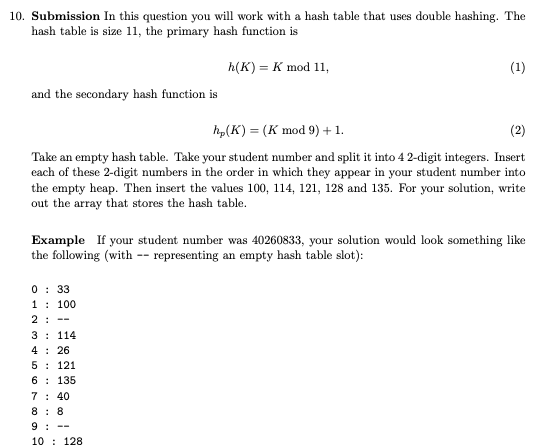10. Submission In this question you will work with a hash table that uses double hashing. The hash table is size 11, the primary hash function is h(K)-K mod 11, and the secondary hash function is hp(K)-(K mod9) +1 Take an empty hash table. Take your student number and split it into 4 2-digit integers. Insert each of these 2-digit numbers in the order in which they appear in your student number into the empty heap. Then insert the values...

• ### 10. (5 points) Consider data with integer keys 28, 21, 11, 47, 36, 19, 32 in...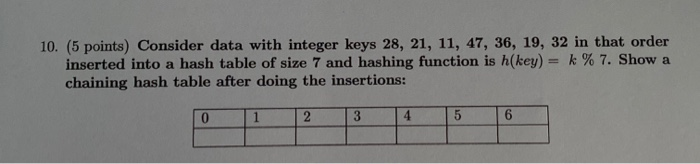10. (5 points) Consider data with integer keys 28, 21, 11, 47, 36, 19, 32 in that order inserted into a hash table of size 7 and hashing function is h(key) = k % 7. Show a chaining hash table after doing the insertions:

• ### 17) 12 points Calculate the keys assigned to each value using the modulus hash h(k) -...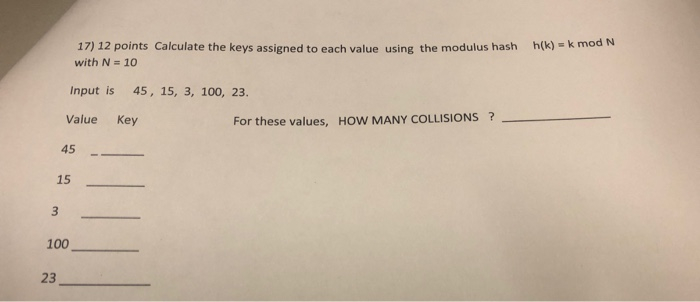17) 12 points Calculate the keys assigned to each value using the modulus hash h(k) - k mod N with N 10 Input is 45, 15, 3, 100, 23. Value Key For these values, HOW MANY COLLISIONS? 45 15 100 23

• ### C++ Problem: Please explain the process! You are given a hash function fl(x) : x %...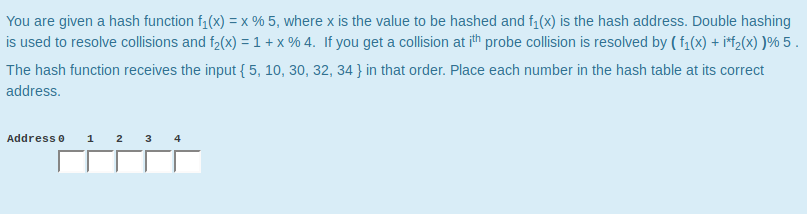C++ Problem: Please explain the process! You are given a hash function fl(x) : x % 5, where x is the value to be hashed and h(x) is the hash address. Double hashing is used to resolve collisions and f2(x)-1 + x 96 4. If you get a collision at ith probe collision is resolved by ( f1(x) + if2(x) )96 5 The hash function receives the input [5, 10, 30, 32, 34 in that order. Place each number in...

• ### 3. (20 points) In open addressing with double hashing, we have h(k,i) hi(k)+ih2(k) mod m, where h...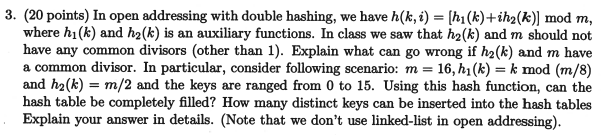3. (20 points) In open addressing with double hashing, we have h(k,i) hi(k)+ih2(k) mod m, where hi(k) and h2(k) is an auxiliary functions. In class we saw that h2(k) and m should not have any common divisors (other than 1). Explain what can go wrong if h2(k) and m have a common divisor. In particular, consider following scenario: m- 16, h(k) k mod (m/8) and h2(k)-m/2 and the keys are ranged from 0 to 15. Using this hash function, can...

• ### Please select file(s) Select file(s) Q9 Double 15 Points Consider inserting the keys 10, 22, 31,...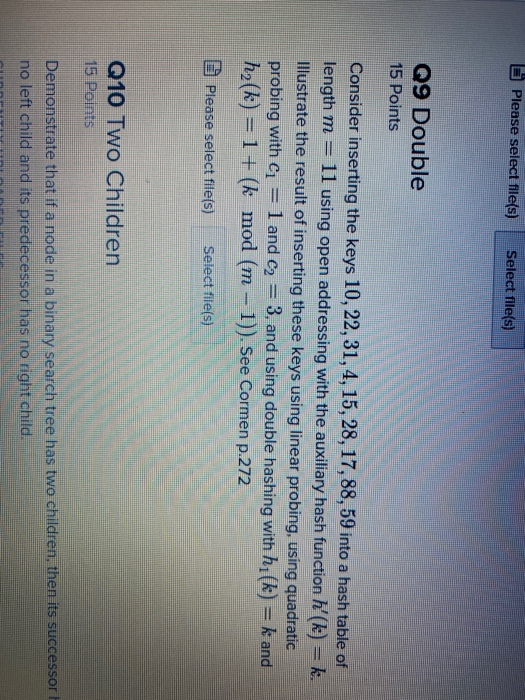Please select file(s) Select file(s) Q9 Double 15 Points Consider inserting the keys 10, 22, 31, 4, 15, 28, 17, 88, 59 into a hash table of length m 11 using open addressing with the auxiliary hash function l'(k) = k. Illustrate the result of inserting these keys using linear probing, using quadratic probing with c1 3, and using double hashing with h1(k) = k and h2(k) = 1 + (k mod (m – 1)). See Cormen p.272 1 and...